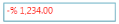# Culture and Formatting in WPF Percent TextBox

21 May 20216 minutes to read

Value of `PercentTextBox` can be formatted in following ways:

• Culture
• NumberFormatInfo
• Dedicated properties (PercentGroupSeparator, PercentGroupSizes, PercentDecimalDigits, PercentDecimalSeparator)

## Culture based formatting

The PercentTextBox provides support for globalization by using the Culture property. The `Culture` property is used to format the decimal separator and group separator of the `PercentTextBox` value based on the respective culture.

``````<syncfusion:PercentTextBox x:Name="percentTextBox" Height="25" Width="150"
Culture="bs-Latn" PercentValue="1234567"/>``````
``````PercentTextBox percentTextBox = new PercentTextBox();
percentTextBox.Width = 150;
percentTextBox.Height = 25;
percentTextBox.PercentValue = 1234567;

//Setting Latin culture for percent textbox.
percentTextBox.Culture = new CultureInfo("bs-Latn");``````

By default the US culture uses “,” as the `PercentGroupSeparator` and “.” as the `PercentDecimalSeparator` where as the Latin culture uses “.” as the `PercentGroupSeparator` and “,” as the `PercentDecimalSeparator`.

Default Culture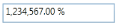Latin Culture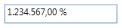## NumberFormatInfo based formatting

The number formatting of `PercentTextBox` can be customized by setting NumberFormat property.

``````<syncfusion:PercentTextBox x:Name="percentTextBox" Height="25" Width="150" PercentValue="1234567">
<syncfusion:PercentTextBox.NumberFormat >
<numberformat:NumberFormatInfo PercentGroupSeparator="/"
PercentSymbol="%"
PercentDecimalDigits="4"
PercentDecimalSeparator="*" />
</syncfusion:PercentTextBox.NumberFormat>
</syncfusion:PercentTextBox>``````
``````PercentTextBox percentTextBox = new PercentTextBox();
percentTextBox.Width = 150;
percentTextBox.Height = 25;
percentTextBox.PercentValue = 1234567;
percentTextBox.NumberFormat = new NumberFormatInfo()
{
PercentGroupSeparator = "/",
PercentDecimalDigits = 4,
PercentDecimalSeparator = "*",
PercentSymbol = "%"
};``````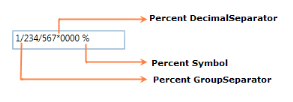The following code illustrate how to set percent group size by using the `NumberFormat` property.

``````PercentTextBox percentTextBox = new PercentTextBox();
percentTextBox.Width = 150;
percentTextBox.Height = 25;
percentTextBox.PercentValue = 123456789;
percentTextBox.NumberFormat = new NumberFormatInfo()
{
PercentGroupSeparator = "/",
PercentDecimalDigits = 4,
PercentDecimalSeparator = "*",
PercentSymbol = "%",

// Adding the Number group size via NumberFormat property.
PercentGroupSizes = new int[] { 2, 3, 4 }
};``````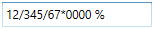## Formatting with dedicated properties

The number formatting of `PercentTextBox` can also be customized by setting the PercentGroupSeparator, PercentGroupSizes, PercentDecimalDigits, PercentDecimalSeparator, `PercentNegativePattern`, `PercentPositivePattern`, and PercentageSymbol properties of PercentTextBox. You can show the group separator by setting the GroupSeperatorEnabled property to `true`.

The following code illustrate how to format using the `PercentDecimalSeparator`, `PercentDecimalDigits`, `PercentGroupSeparator`, `PercentGroupSizes` property of the `PercentTextBox`.

``````PercentTextBox percentTextBox = new PercentTextBox();
percentTextBox.Width = 150;
percentTextBox.Height = 25;
percentTextBox.PercentValue = 123456789;
percentTextBox.GroupSeperatorEnabled = true;
percentTextBox.PercentageSymbol = "%";
percentTextBox.PercentDecimalDigits = 4;
percentTextBox.PercentDecimalSeparator = "/";
percentTextBox.PercentGroupSeparator = "*";

// Adding the percent group size via NumberGroupSizes property.
percentTextBox.PercentGroupSizes = new Int32Collection() { 4, 3, 2};``````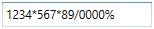NOTE

When you use both the `NumberFormat` and the dedicated properties (`PercentGroupSeparator`, `PercentageSymbol`, `PercentDecimalDigits`, `PercentDecimalSeparator` and `PercentGroupSizes`) to format the value of `PercentTextBox`, the `PercentGroupSeparator`and `PercentGroupSizes` properties have higher priority.

NOTE

When you use both `NumberFormat` and `Culture`, the `NumberFormat` will have a higher priority.

## Positive Value Pattern

You can use the PercentPositivePattern property to customize the location of the percent symbol and the positive percent values. In the table below,”%” denotes the symbol of the percent, and “n” denotes the number.

PercentPositivePattern table

Value Associated Pattern
0 n %
1 n%
2 %n
3 % n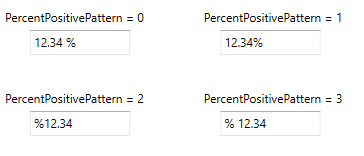``````<syncfusion:PercentTextBox x:Name="percentTextBox" Height="25" Width="150"
PercentValue="1234" PercentPositivePattern="3"/>``````
``````PercentTextBox percentTextBox = new PercentTextBox();
percentTextBox.Width = 150;
percentTextBox.Height = 25;
percentTextBox.Value = 1234;
percentTextBox.PercentPositivePattern = 3;``````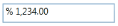## Negative Value Pattern

You can use the PercentNegativePattern property to customize the location of the percent symbol and the negative percent values. In the table below,”%” denotes the symbol of the percent, and “n” denotes the number.

PercentNegativePattern table

Value Associated Pattern
0 -n %
1 -n%
2 -%n
3 %-n
4 %n-
5 n-%
6 n%-
7 -% n
8 n %-
9 % n-
10 % -n
11 n- %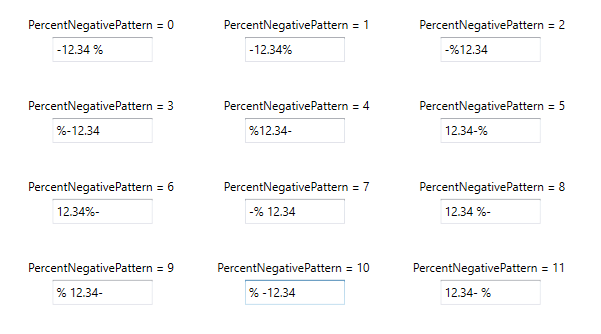``````<syncfusion:PercentTextBox x:Name="percentTextBox" Height="25" Width="150"
PercentValue="1234" PercentNegativePattern="7"/>``````
``````PercentTextBox percentTextBox = new PercentTextBox();
percentTextBox.Width = 150;
percentTextBox.Height = 25;
percentTextBox.PercentValue = 1234;
percentTextBox.PercentNegativePattern = 7;``````